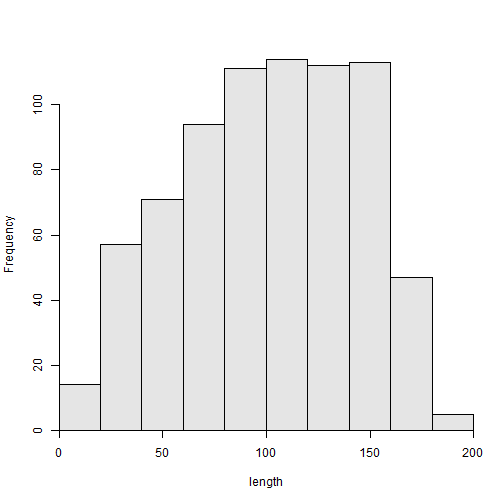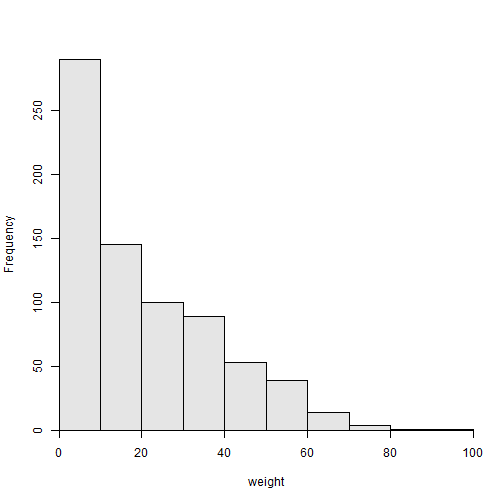## The Problem

I have been attempting to use RMarkdown rather than LaTeX to produce R examples. One issue that has slowed my conversion has been my struggles with how to reference figures and tables. The examples below illustrate how I have been using `captioner` to solve this problem.

## The Solution

### Foundation

For a simple example, I load `FSAdata` for the `RuffeSLRH92` data, `FSA` for `hist()` and `summarize()`, and `knitr` for `kable` (to produce an RMarkdown table). Additionally, `captioner` is loaded.

Separate `captioner` objects must be initialized for handling figures and tables. The `prefix=` argument sets the common prefix for all items of a certain type. Below, I initialize a function for creating captoins for figures and tables. The result of this code is two functions – one called `figs` that will hold a list of tags and captions for figures and another called `tbls` that will hold the same for tables.

These functions can be used to create on object that holds a tag, caption, and number for figures or tables, respectively. Initially, these functions are called with two arguments – the figure or table tag and the figure or table caption. For example, the code below creates tag and caption combinations for two figures. I prefer to create all tags and captions in one chunk (and use `results='hide'` to hide the immediate display of the information).

The same function may then be used to retrieve the function name with a number, the figure number with a prefix, or the figure number.

The results returned by this function are exploited, as shown in the next section, to solve the figure referencing problem.

### Application

The `figs` function may be used to add a figure caption to a figure. For example, the chunk below is followed by an inline R chunk of Figure 1: Length frequency of Ruffe captured in 1992..Figure 1: Length frequency of Ruffe captured in 1992.

In addition, use inline R code to refer to the figure. For example, `figs("LenFreq1",display="cite")` inside an inline R call would produce a reference to Figure 1.

As more functions are added, the figure numbers are incremented such that inline R code may refer to Figure 2 and Figure 1.Figure 2: Weight frequency of Ruffe captured in 1992.

Tables can be handled in the same way. For example, see Table 1, which is related to Figure 1 and Figure 2.

Table 2: Summary statistics of the length and weight of Ruffe captured in 1992.